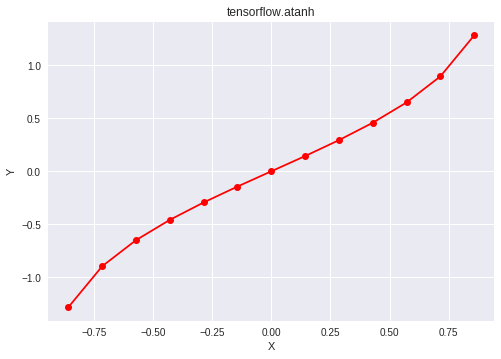# Python | Tensorflow atanh() method

• Last Updated : 10 Nov, 2021

Tensorflow is an open-source machine learning library developed by Google. One of its applications is to develop deep neural networks.
The module tensorflow.math provides support for many basic mathematical operations. Function tf.atanh() [alias tf.math.atanh] provides support for the inverse hyperbolic tangent function in Tensorflow. Its domain is in the range [-1, 1] and it returns nan for any input outside this range. The input type is tensor and if the input contains more than one element, element-wise inverse hyperbolic tangent is computed.

Syntax: tf.atanh(x, name=None) or tf.math.atanh(x, name=None)
Parameters
x: A tensor of any of the following types: float16, float32, float64, complex64, or complex128.
name (optional): The name for the operation.
Return type: A tensor with the same type as that of x.

Attention geek! Strengthen your foundations with the Python Programming Foundation Course and learn the basics.

To begin with, your interview preparations Enhance your Data Structures concepts with the Python DS Course. And to begin with your Machine Learning Journey, join the Machine Learning - Basic Level Course

Code #1:

## Python3

 `# Importing the Tensorflow library``import` `tensorflow as tf``  ` `# A constant vector of size 6``a ``=` `tf.constant([``1.0``, ``-``0.5``, ``-``1``, ``2.4``, ``0.0``, ``-``6.5``], dtype ``=` `tf.float32)``  ` `# Applying the atanh function and``# storing the result in 'b'``b ``=` `tf.atanh(a, name ``=``'atanh'``)``  ` `# Initiating a Tensorflow session``with tf.Session() as sess:``    ``print``(``'Input type:'``, a)``    ``print``(``'Input:'``, sess.run(a))``    ``print``(``'Return type:'``, b)``    ``print``(``'Output:'``, sess.run(b))`

Output:

```Input type: Tensor("Const_3:0", shape=(6, ), dtype=float32)
Input: [ 1.  -0.5 -1.   2.4  0.  -6.5]
Return type: Tensor("atanh_1:0", shape=(6, ), dtype=float32)
Output: [        inf -0.54930615        -inf         nan  0.                 nan]```

Code #2: Visualization

## Python3

 `# Importing the Tensorflow library``import` `tensorflow as tf`` ` `# Importing the NumPy library``import` `numpy as np`` ` `# Importing the matplotlib.pyplot function``import` `matplotlib.pyplot as plt`` ` `# A vector of size 15 with values from -1 to 1``a ``=` `np.linspace(``-``1``, ``1``, ``15``)`` ` `# Applying the inverse hyperbolic tangent``# function and storing the result in 'b'``b ``=` `tf.atanh(a, name ``=``'atanh'``)`` ` `# Initiating a Tensorflow session``with tf.Session() as sess:``    ``print``(``'Input:'``, a)``    ``print``(``'Output:'``, sess.run(b))``    ``plt.plot(a, sess.run(b), color ``=` `'red'``, marker ``=` `"o"``)``    ``plt.title(``"tensorflow.atanh"``)``    ``plt.xlabel(``"X"``)``    ``plt.ylabel(``"Y"``)`` ` `    ``plt.show()`

Output:

```Input: [-1.         -0.85714286 -0.71428571 -0.57142857 -0.42857143 -0.28571429
-0.14285714  0.          0.14285714  0.28571429  0.42857143  0.57142857
0.71428571  0.85714286  1.        ]
Output: [       -inf -1.28247468 -0.89587973 -0.64964149 -0.45814537 -0.29389333
-0.14384104  0.          0.14384104  0.29389333  0.45814537  0.64964149
0.89587973  1.28247468         inf]```My Personal Notes arrow_drop_up